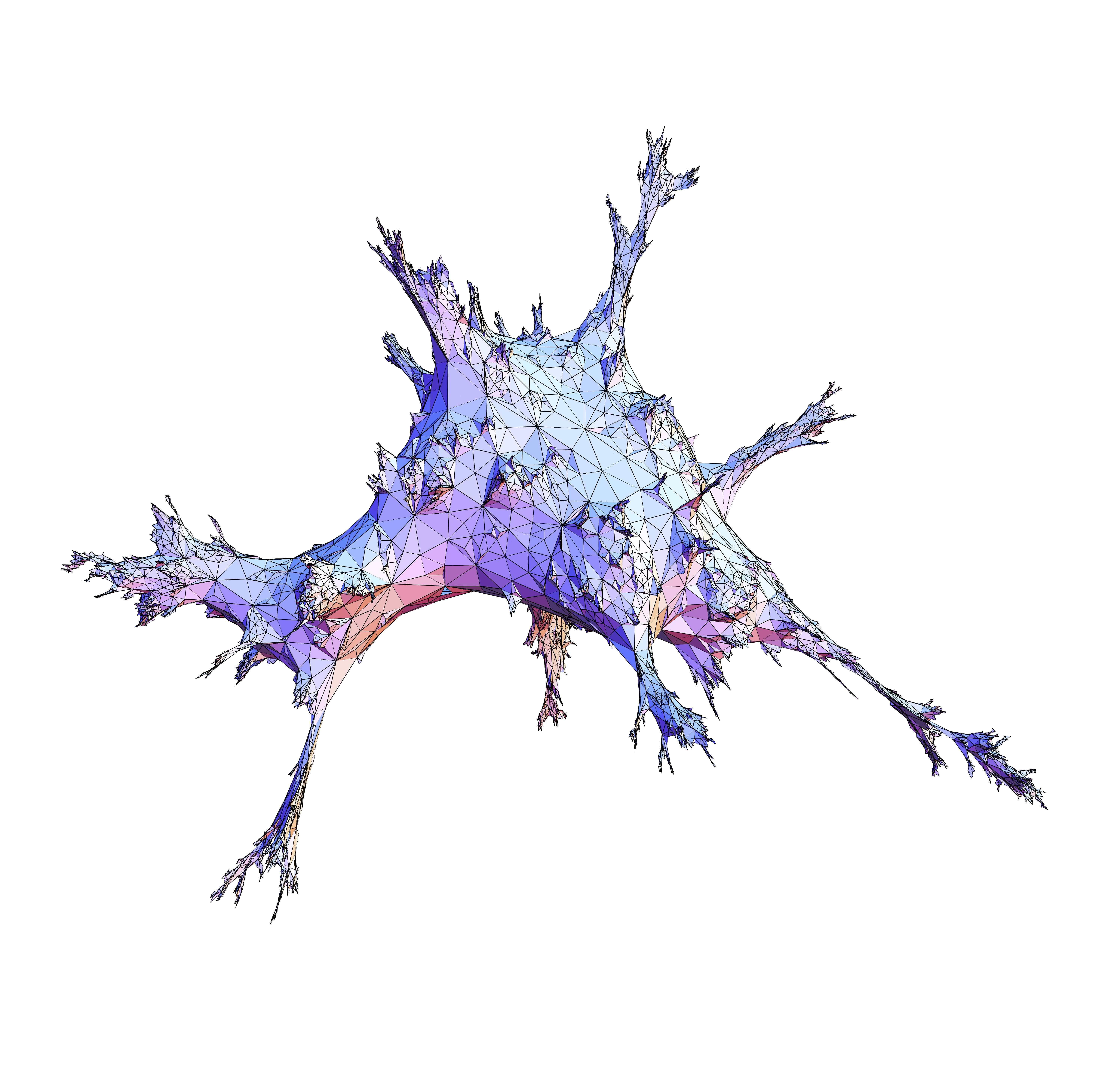Project GEOBROWN funded by the ERC Advanced Grant 740943

# Home Members Publications Events

 Brownian geometry: at the interface between probability theory, combinatorics and physics The main purpose of this project is to explore the canonical models of planar random geometry that have been introduced in the recent years. We call this theory Brownian geometry because one of the central objects, the Brownian map, arises as the universal scaling limit of many discrete models of large random graphs embedded in the plane, in a way very similar to Brownian motion, which is the continuous limit of many different classes of random paths. The main objectives of the project are to combine the different approaches in order to develop a systematic study of the Brownian map and its variants called the Brownian disk and the Brownian plane, as well as of the associated discrete models, which are finite graphs embedded in the plane or infinite random lattices such as the uniform infinite planar triangulation. It is also planned to study random phenomena in random geometry, starting with random walks on infinite random lattices, with the ultimate goal of constructing Brownian motion on our continuous models. A question of importance in mathematical physics is to understand the behavior of statistical physics models in random geometry. The field of random geometry gives rise to exceptionally fruitful interactions between specialists of probability theory, theoretical physicists and mathematicians coming from other areas, in particular from combinatorics.The proposed research relies on the expertise of several members of the Laboratoire de Mathématiques d'Orsay, and on the unique environment of Université Paris-Sud and neighboring institutions.Simulation of a large triangulation